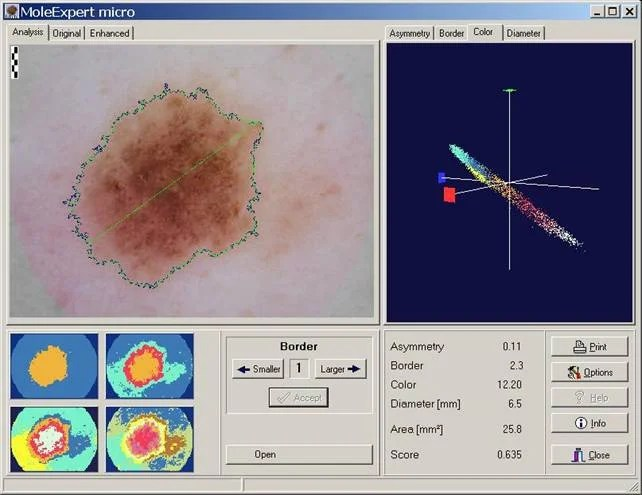## MoleExpert micro softwareThe MoleExpert software is a product is based on experiences of many years with the automated analysis of pigmented skin lesions. Essential requirement with this software project was the usefulness of the software with the most different photograph systems. Qualitatively high-quality, evenly and well illuminated top illumination-microscopic pictures of the lesions is the most essential condition for the operability of this software. MoleExpert micro was developed for the support of diagnostic identification. The system spends no diagnosis for this reason, but supplies as results of measurement data to asymmetry, for the delimitation of the lesion, to the color and the size. These parameters of the ABCD rule are recognized for some years as important dermatoscopic parameters. According to a particular algorithm adapted on the image analysis, the four ABCD values are combined into a total core, which can take values between zero to unify. With lesions with high Score, it acts with higher probability around a Melanoma, than with lesions with low Score. Download demo version from here: MoleExpert micro

## Binary watch with LED matrix display

In this video, the guy managed to make a binary wristwatch with an LED display. All settings are accessible through a scrolling menu system on its 3×4 LED matrix. Watch also include voltage meter, binary counter, club mode, and time display.

## Frequency response of discrete systemIn previous post we discussed about impulse response. Impulse response h(n) is digital system response in time domain. But there is another characterization of discrete system – frequency response H(ejω). Frequency response can be calculated form impulse response by formula: This means frequency response is systems transfer coefficient to every frequency value. Frequency response is a complex function. So this can be evaluated as:

## Digital system equationBack to DSP thread. Lets talk about digital system equation. Every digital system can be described using this equation. The output of digital filter generally consist of previous inputs and previous outputs. y(n-k) is considered as previous outputs; x(n-p) – previous inputs; ak and b­p – coefficients; This equation is convenient to define discrete system and extract various characteristics. Number N defines discrete filter tap (Tap – A FIR “tap” is simply a coefficient/delay pair). Lets say we have digital filter made of one tap. y(n)=a·y(n-1)+b·x(n) First we calculate filter response function – h(n): Response function is calculated from system reaction to discrete impulse ´(n). So assume that x(n)= ´(n) and y(n)=h(n). Initial conditions: y(-1)=h(-1)=0. Then: h(n)=0 when n<0; h(0)=a·h(-1)+b·´(n)=b; h(1)=a·h(0)+b·´(1)=ab; ……. h(n)= ban where n>0 Lets say we have a=0.7; b=1; Then we get discrete system characteristics: This equation in example describes IIR (Infinite Impulse Response) discrete systems, because there is a feedback element a·y(n-1). There is another type of discrete filter – FIR (Finite Impulse Response) Finite response filter equation is much simpler: As you may noticed from equation – IIR filter have infinite number of response impulses while FIR filter have a finite number of impulses. IIR…

## AVR microcontroller interrupts using WINAVR